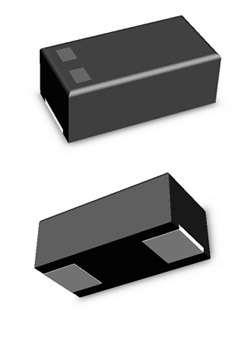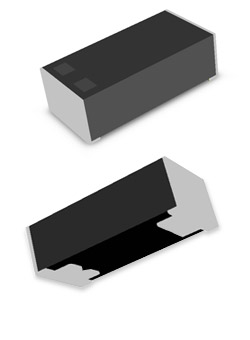## Tantalum

Part Number
Status
Use For
Features
Capacitance
~
Tolerance
Rated Vdc
Vdc
~
Vdc
Vdc
ESR (100kHz)
mΩ
~
mΩ
mΩ
Size Code(mm/inch)
L Size
W Size
T Size

### Search Result

* Please click the search button above to see results.
Matching Parts
Total Parts
20

### Part Numbering Guide

1. 1
TC
Tantalum
Capacitor
2. 2
PCF
Series
3. 3
0J
Rated
Voltage
4. 4
476
Capacitance
5. 5
M
Tolerance
6. 6
R
Size
7. 7
A
Packing
8. 8
R
Packing
Polarity
9. 9
0150
ESR
In Milliohms
1. 1TCPCFOJ476MRAR0150

#### TANTALUM CAPACITOR CODE

TC = Tantalum Capacitor

2. 2TCPCFOJ476MRAR0150

#### SERIES CODE

3. 3TCPCFOJ476MRAR0150

#### RATED VOLTAGE CODE

• 0E = 2.5V
• 0G = 4V
• 0J = 6.3V
• 1A = 10V
• 1C = 16V
• 1D = 20V
• 1E = 25V
• 1V = 35V
4. 4TCPCFOJ476MRAR0150

#### CAPACITANCE CODE

• 104 = 0.1㎌
• 105 = 1.0㎌
• 476 = 47㎌
• 107 = 100㎌

The first and second figures mean capacitance (unit: pF),
the third figure expresses the number of zeros.
Example) 106 = 10pF X 106 = 10㎌

5. 5TCPCFOJ476MRAR0150

#### TOLERANCE CODE

• K = ±10%
• M = ±20%
6. 6TCPCFOJ476MRAR0150

#### SIZE CODE

• J = 1608
• P/R/N/O = 2012
• A = 3216
• B/T = 3528
• C = 6032
• D = 7343
7. 7TCPCFOJ476MRAR0150

#### PACKING CODE

• A = 7 inch
• C = 13 inch
8. 8TCPCFOJ476MRAR0150

#### PACKING POLARITY CODE

• R = Reel Type
• B = Bulk Type
9. 9TCPCFOJ476MRAR0150

#### ESR IN MILLIOHMS CODE

• 0009 = 9mΩ
• 0035 = 35mΩ
• 0150 = 150mΩ
• 0300 = 300mΩ
• 0500 = 500mΩ

### Features Description

• · PCM Series• · PCF/PBL Series• · PFT Series